Home | | Maths 12th Std | Euler‚Äôs Form of the complex number

# Euler‚Äôs Form of the complex number

When performing multiplication or finding powers or roots of complex numbers, Euler form can also be used.

Euler‚Äôs Form of the complex number

The following identity is known as Euler‚Äôs formula

eiŒ∏  = cosŒ∏ + i sinŒ∏

Euler formula gives the polar form z = r eiŒ∏

Note

When performing multiplication or finding powers or roots of complex numbers, Euler form can also be used.

Example 2.22

Find the modulus and principal argument of the following complex numbers.

(i) ‚àö3 + i

(ii) -‚àö3 + i

(iii) - ‚àö3 - i

(iv) ‚àö3 - i

Solution

(i) ‚àö3 + i

Modulus =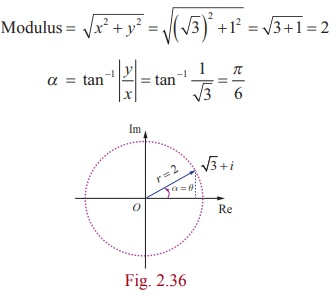Since the complex number has the principal value ‚àö3 + i lies in the first quadrant, has the principal value

Œ∏ = Œ± = œÄ/6.

Therefore, the modulus and principal argument of ‚àö3 + i are 2 and œÄ/6 respectively.

(ii) -‚àö3 + i

Modulus = 2 andSince the complex number  -‚àö3 + i lies in the second quadrant has the principal valueTherefore the modulus and principal argument of (ii) -‚àö3 + i are 2 and 5œÄ/6 respectively.

(iii) - ‚àö3 - i

r = 2 and Œ± = œÄ /6 .

Since the complex number - ‚àö3 - i lies in the third quadrant, has the principal value,Therefore, the modulus and principal argument of - ‚àö3 - i are 2 and -5œÄ/6 respectively.

(iv) ‚àö3 - i

r = 2 and Œ± = œÄ/6

Since the complex number lies in the fourth quadrant, has the principal value,

Œ∏ = -Œ± = -œÄ/6Therefore, the modulus and principal argument of

‚àö3 - i  are 2 and - œÄ/6 .

In all the four cases, modulus are equal, but the arguments are depending on the quadrant in which the complex number lies.

Example 2.23

Represent the complex number (i) -1- i (ii) 1+ i‚àö3 in polar form.

Solution

(i) Let -1- i = r(cosŒ∏ + isinŒ∏ )

We have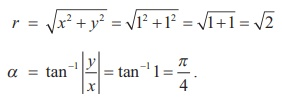Since the complex number -1- i lies in the third quadrant, it has the principal value,Note

Depending upon the various values of k , we get various alternative polar forms.

(ii) 1+ i‚àö3Therefore, the polar form of 1+ i‚àö3 can be written as### Example 2.24

Find the principal argument Arg z , when z = -2 / [ 1+i‚àö3 ].

### SolutionThis implies that one of the values of arg z is 2œÄ/3 .

Since 2œÄ/3 lies between -œÄ and œÄ, the principal argument Arg z is 2 œÄ/3.

## Properties of polar form

### Property 1

If z = r (cosŒ∏ + i sinŒ∏ ), then z-1 =1/r  (cosŒ∏ - i sinŒ∏ ) .

### Proof### Property 2

If z1  = r1 (cosŒ∏1  + i sinŒ∏1 ) and  z2  = r2 (cosŒ∏2  + i sinŒ∏2 ) ,

then   z1 z2  = r1r2 (cos (Œ∏1  + Œ∏2 ) + i sin (Œ∏1  + Œ∏2 )) .

### Proof

z = r (cosŒ∏1 + i sinŒ∏1) and

= r (cosŒ∏2  + i sinŒ∏2)‚áí z1 z2   =  r1 (cosŒ∏1  + i sinŒ∏1 ) r2 (cosŒ∏2  + i sinŒ∏2 )

r1r2 ((cosŒ∏1 cosŒ∏2  - sinŒ∏1 sinŒ∏2 ) + i (sinŒ∏1 cosŒ∏2  + sinŒ∏2  cosŒ∏1 ))

z1 z2   =  r1r2 (cos (Œ∏1  + Œ∏2 ) + i sin (Œ∏1  + Œ∏2 )) .

### Note

arg ( z1 z2 ) = Œ∏1 + Œ∏2 = arg ( z1 ) + arg ( z2 ) .

### Property 3Proof

Using the polar form of z1 and z2, we have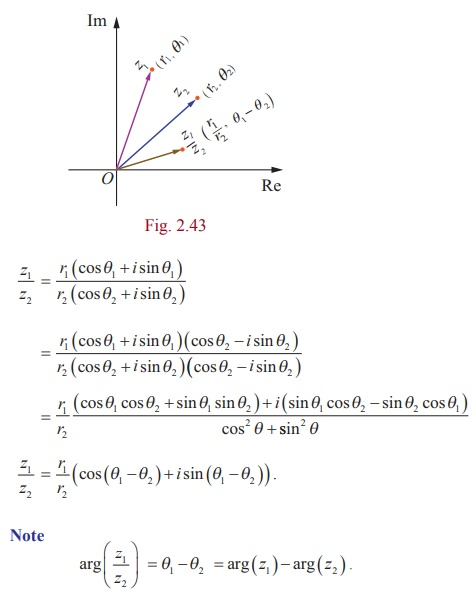Example 2.25

Find the productin rectangular from.

Solution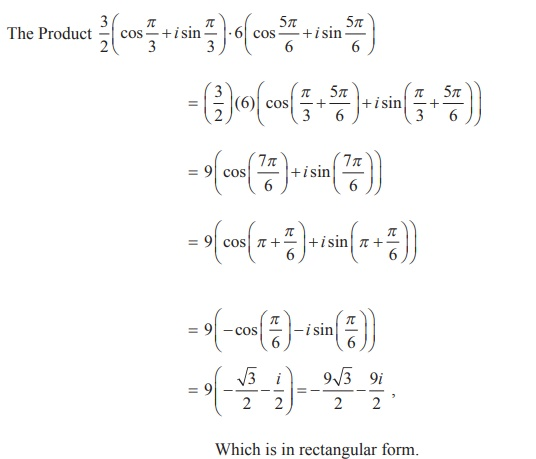Example 2.26

Find the quotientin rectangular form.

SolutionWhich is in rectangular form.

Example 2.27

If z = x + iy and, show that x2 + y2 =1.

Solution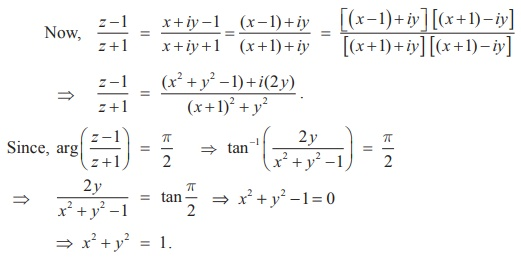‚áí x2 + y2 =1

Tags : Definition, Properties, Formulas, Solved Example Problems , 12th Mathematics : UNIT 2 : Complex Numbers
Study Material, Lecturing Notes, Assignment, Reference, Wiki description explanation, brief detail
12th Mathematics : UNIT 2 : Complex Numbers : Euler‚Äôs Form of the complex number | Definition, Properties, Formulas, Solved Example Problems